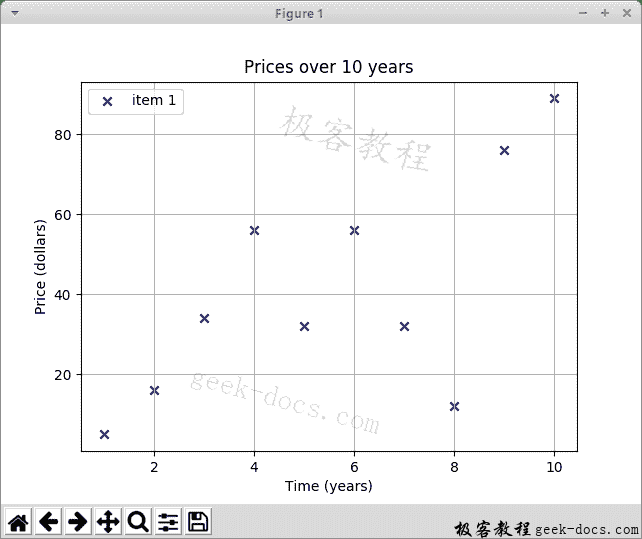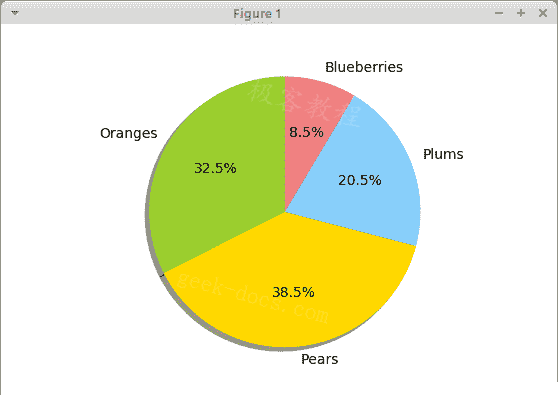# Matplotlib入门指南

## Matplotlib

Matplotlib 是用于创建图表的 Python 库。 Matplotlib 可用于 Python 脚本，Python 和 IPython shell，jupyter 笔记本，Web 应用服务器以及四个图形用户界面工具包。

## Matplotlib 安装

Matplotlib 是需要安装的外部 Python 库。

``````\$ sudo pip install matplotlib
``````
Py

## Matplotlib 散点图

`scatter.py`

``````#!/usr/bin/python3

import matplotlib.pyplot as plt

x_axis = [1, 2, 3, 4, 5, 6, 7, 8, 9, 10]
y_axis = [5, 16, 34, 56, 32, 56, 32, 12, 76, 89]

plt.title("Prices over 10 years")
plt.scatter(x_axis, y_axis, color='darkblue', marker='x', label="item 1")

plt.xlabel("Time (years)")
plt.ylabel("Price (dollars)")

plt.grid(True)
plt.legend()

plt.show()
``````
Py

``````import matplotlib.pyplot as plt
``````
Py

``````x_axis = [1, 2, 3, 4, 5, 6, 7, 8, 9, 10]
y_axis = [5, 16, 34, 56, 32, 56, 32, 12, 76, 89]
``````
Py

``````plt.title("Prices over 10 years")
``````
Py

``````plt.scatter(x_axis, y_axis, color='darkblue', marker='x', label="item 1")
``````
Py

`scatter()`功能绘制散点图。 它接受 x 和 y 轴，标记的颜色，标记的形状和标签的数据。

``````plt.xlabel("Time (years)")
plt.ylabel("Price (dollars)")
``````
Py

``````plt.grid(True)
``````
Py

``````plt.legend()
``````
Py

`legend()`功能在轴上放置图例。

``````plt.show()
``````
Py

`show()`功能显示图表。## 两个数据集

`scatter2.py`

``````#!/usr/bin/python3

import matplotlib.pyplot as plt

x_axis1 = [1, 2, 3, 4, 5, 6, 7, 8, 9, 10]
y_axis1 = [5, 16, 34, 56, 32, 56, 32, 12, 76, 89]

x_axis2 = [1, 2, 3, 4, 5, 6, 7, 8, 9, 10]
y_axis2 = [53, 6, 46, 36, 15, 64, 73, 25, 82, 9]

plt.title("Prices over 10 years")

plt.scatter(x_axis1, y_axis1, color='darkblue', marker='x', label="item 1")
plt.scatter(x_axis2, y_axis2, color='darkred', marker='x', label="item 2")

plt.xlabel("Time (years)")
plt.ylabel("Price (dollars)")

plt.grid(True)
plt.legend()

plt.show()
``````
Py

``````x_axis1 = [1, 2, 3, 4, 5, 6, 7, 8, 9, 10]
y_axis1 = [5, 16, 34, 56, 32, 56, 32, 12, 76, 89]

x_axis2 = [1, 2, 3, 4, 5, 6, 7, 8, 9, 10]
y_axis2 = [53, 6, 46, 36, 15, 64, 73, 25, 82, 9]
``````
Py

``````plt.scatter(x_axis1, y_axis1, color='darkblue', marker='x', label="item 1")
plt.scatter(x_axis2, y_axis2, color='darkred', marker='x', label="item 2")
``````
Py

## Matplotlib 折线图

`linechart.py`

``````#!/usr/bin/python3

import numpy as np
import matplotlib.pyplot as plt

t = np.arange(0.0, 3.0, 0.01)
s = np.sin(2.5 * np.pi * t)
plt.plot(t, s)

plt.xlabel('time (s)')
plt.ylabel('voltage (mV)')

plt.title('Sine Wave')
plt.grid(True)

plt.show()
``````
Py

``````import numpy as np
``````
Py

``````t = np.arange(0.0, 3.0, 0.01)
``````
Py

`arange()`函数返回给定间隔内的均匀间隔的值列表。

``````s = np.sin(2.5 * np.pi * t)
``````
Py

``````plt.plot(t, s)
``````
Py

## Matplotlib 条形图

`barchart.py`

``````#!/usr/bin/python3

from matplotlib import pyplot as plt
from matplotlib import style

style.use('ggplot')

x = [0, 1, 2, 3, 4, 5]
y = [46, 38, 29, 22, 13, 11]

fig, ax = plt.subplots()

ax.bar(x, y, align='center')

ax.set_title('Olympic Gold medals in London')
ax.set_ylabel('Gold medals')
ax.set_xlabel('Countries')

ax.set_xticks(x)
ax.set_xticklabels(("USA", "China", "UK", "Russia",
"South Korea", "Germany"))

plt.show()
``````
Py

``````style.use('ggplot')
``````
Py

``````fig, ax = plt.subplots()
``````
Py

`subplots()`函数返回图形和轴对象。

``````ax.bar(x, y, align='center')
``````
Py

``````ax.set_xticks(x)
ax.set_xticklabels(("USA", "China", "UK", "Russia",
"South Korea", "Germany"))
``````
Py

## Matplotlib 饼图

`piechart.py`

``````#!/usr/bin/python3

import matplotlib.pyplot as plt

labels = ['Oranges', 'Pears', 'Plums', 'Blueberries']
quantity = [38, 45, 24, 10]

colors = ['yellowgreen', 'gold', 'lightskyblue', 'lightcoral']

plt.pie(quantity, labels=labels, colors=colors, autopct='%1.1f%%',

plt.axis('equal')

plt.show()
``````
Py

``````labels = ['Oranges', 'Pears', 'Plums', 'Blueberries']
quantity = [38, 45, 24, 10]
``````
Py

``````colors = ['yellowgreen', 'gold', 'lightskyblue', 'lightcoral']
``````
Py

``````plt.pie(quantity, labels=labels, colors=colors, autopct='%1.1f%%',
``````
Py

``````plt.axis('equal')
``````
Py### 觉得文章有用就打赏一下文章作者

#### 支付宝扫一扫打赏#### 微信扫一扫打赏• 回顶